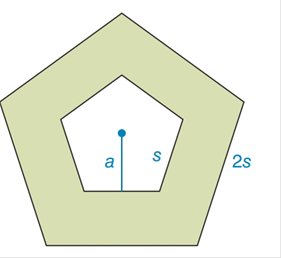Chapter 8.3, Problem 32EElementary Geometry For College St...

7th Edition
Alexander + 2 others
ISBN: 9781337614085

Solutions

Chapter
SectionElementary Geometry For College St...

7th Edition
Alexander + 2 others
ISBN: 9781337614085
Textbook Problem

In terms of the apothem a and length of side s of the smaller regular pentagon, find an expression for the shaded area.To determine

To find:

An expression for the shaded area in given pentagon.

Explanation

1) The perimeter of a regular polygon is given by P = ns, where n is the number of sides and s is the length of any side.

2) The area of a regular polygon with apothem a and perimeter P is given by A=12(aP).

3) If the side of the regular polygon in s then the apothem a is

a=s2tan(180n), where n is the number of sides.

Calculation:

Given,

The smaller regular pentagon with side s and apothem a and the large regular pentagon with side 2s.

Then the area of the shaded portion is equal to the area of the larger regular pentagon - area of the smaller pentagon.

To find the area of the smaller pentagon

Use the formula for the perimeter of regular polygon, i.e., perimeter = ns

Substitute n = 5 and s = s in P = ns.

P=5s

Use the formula of area of regular pentagon, A=12(aP)

Substitute a = a and P = 5s in A=12(aP).

A=12(a5s)=52as=2.5as

The area of the smaller pentagon is 2.5as

Still sussing out bartleby?

Check out a sample textbook solution.

See a sample solution

The Solution to Your Study Problems

Bartleby provides explanations to thousands of textbook problems written by our experts, many with advanced degrees!

Get Started

Find more solutions based on key concepts